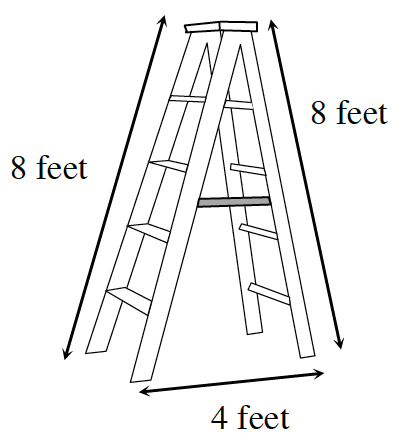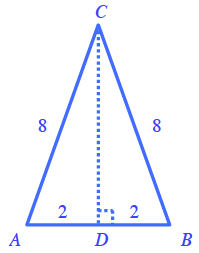### Home > GC > Chapter 11 > Lesson 11.1.5 > Problem11-66

11-66.

Hokiri’s ladder has two legs that are each $8$ feet long. When the ladder is opened safely and locked for use, the legs are $4$ feet apart on the ground. What is the angle that is formed at the top of the ladder where the legs meet?Create a diagram of the ladder with a perpendicular bisector from the top ($∠C$) to the base ($\overline{AB}$).

Next, use the right triangle $ADC$ and a calculator to find the angle whose sine is equal to $\frac{2}{8}$.

$\text{sin}\left(\frac{m\angle ACD}{2}\right)=\frac{2}{8}$ or $\frac{m\angle ACD}{2}=\text{arcsin}\left(\frac{2}{8}\right)$

Since $\angle ACD$ is half of the desired angle, double $m\angle ACD$ to get $m∠ACB$.

$m∠ACB=29°$